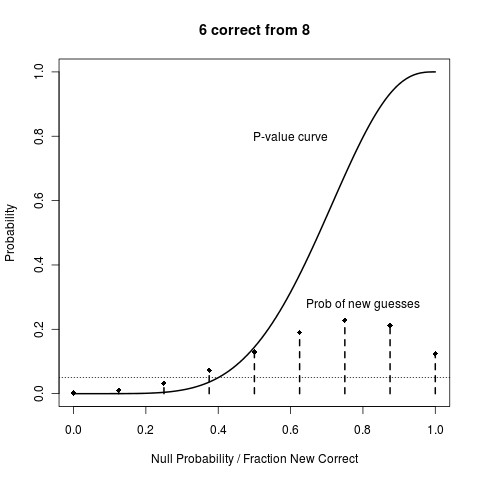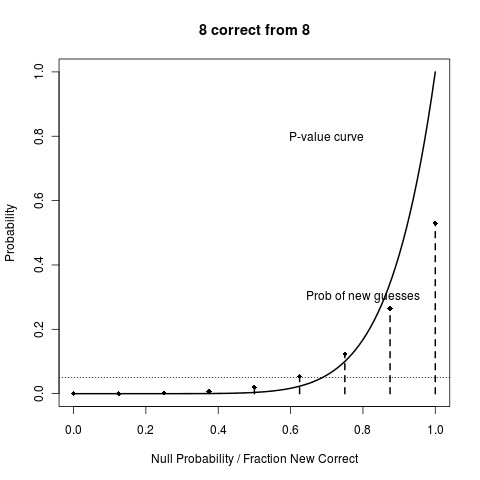Statistics

# Supplement For Your Tea

This is meant to clear up some confusion in the Lady Tasting Tea series, which featured a problem people hoped would be easy.

Here are two possible outcomes, comparing the frequentist hypothesis testing with the Bayesian agnostic model, where the agnostic model is one which we say we have no idea in advance what fraction of cups she will guess correctly, except that we have knowledge that there will be 8 cups (we don’t even say we know how many will be milk-first and how many tea-first).

The frequentist p-value is:

(4) Pr(T(M,8) > t(M,8) | Null hypothesis of what has the ability means)

and where M equals either 6 or 8 correct guesses (there are conflicting reports how many cups she got right). The solid curve in the figure below changes the the “null hypothesis” from 0 to 1, meaning it is the null hypothesis that “has no ability” means the probability of guessing any cup correctly is p.

The solid curve shows that the p-value (4) changes for every p. A helpful dotted line has been drawn for the magic value. So if the lady guessed 6 correctly, we would have “rejected the null” whenever the null was p = 0.4 or below.We already know—we have deduced—that if “has the ability” meant “she guesses all correctly” that the this model is false. If we said “has the ability” mean “she guesses at least N/2 correctly”, and we had no other interpretations in contention, then this the probability this model is true remains 1.

But if we allow agnosticism in the way mentioned above, then we are not going to say after the first N = 8 cups whether she “has the ability” or not. Instead, we will use the evidence from the first experiment to suggest she has a certain ability, which, given the evidence, says she will get about 6 out of every 8 new cups right.

Suppose we were to guess, given this agnostic model and the evidence of the experiment and the data from the experiment itself, what the probability she will guess M’ new cups out of N’. That’s what the spikes in the second curve are, for N’ = 8: these spikes are at the points M’/N’, M’ = 0, 1, 2,…,8, so that they can be compared with the frequentist answer.

Notice particularly that the most likely number of new cups (given the old information) is M’ = 7. It is not M’ = 6.

The next picture is the same thing, except assuming she originally guessed M = N = 8. We would now reject all “nulls” that are about p = 0.7 or smaller.The number of new cups M’ has shifted to higher probabilities of larger numbers of correct guesses, as we might expect. But even thought she got all right before, notice there is still a good chance that she won’t get all right in the next 8. There is just greater than a 50% chance that she will, but it is not certain that she will.

The frequentist answer, for all null hypotheses under p = 0.7, would be to say that she would guess all future numbers of cups perfectly, no matter how many new cups there will be. Why? Because we have rejected any hypothesis which calls for less-than-perfect probabilities. Indeed, the frequentist estimate for p is M/N = 1.

Pause here and reflect until this last point seeps in. It is crucial.

Here is why frequentist procedure sends one off more confident than one should be. Again, we are looking at the probability of guessing correctly M’/N’ new cups, but only for when the old evidence was she guessed 6 out of 8 correctly (if you understood everything, you know why I needn’t craw the corresponding 8 out of 8 picture).The black lines are the frequentist guess using the plug-in estimator p = 6/8. The blue lines are the same as they were in the first picture: the result of applying the agnostic model to new data. Notice that the Bayesian answer is more spread out, i.e. more uncertain.

And remember! This line only holds for the specific agnostic model given. If you really do mean that “has the ability” means “guessing all correctly no matter what” then we know, if M = 6, that this model is false, i.e. that she does not have the ability.

I will be away from the computer until Friday.

Categories: Statistics

### 3 replies »

1.mt says:

“We already knowâ€”we have deducedâ€”that if â€œhas the abilityâ€ meant â€œshe guesses all correctlyâ€
“we had no other interpretations in contention”

These are what I want to focus on, and just looking at the Bayesian result, just looking at the result of the one experiment.

P = “she has the ability to differentiate milk-first and tea-first by taste”
Q = “she guesses all cups correctly in our experiment”

When we start the experiment, we say “if P then Q”. Q is arbitrary, it could be 7 correct of 8 guesses or 6 of 8 or whatever, but it’s the rule we set. My issue is that the Bayesian seems to be saying, “I saw Q, therefore P” (a.k.a. Pr(P|Q)=1). That doesn’t logically follow, because there are other conditions that could have lead to Q (luck, cheating, ESP [after all, this wasn’t a double blind experiment]).

Does “no other interpretations in contention” mean that we accept no other Q as a valid rule, or that we’re assuming that nothing else but ability could lead to a correct call? What is it that allows the Bayesian to ignore other sources of correct guesses? Whatever it is, it should be part of E in Pr(ability|E)=1, so that it’s explicitly defined. If there’s nothing, than the Bayesian is overconfident in his result.

2.mt says:

Something is strange about the frequentist results. For the 8 correct from 8 guesses result, the null hypothesis is rejected when p < 0.7, therefore she will always guess correctly. For the 6 correct from 8 guesses result, the null hypothesis is rejected when p < 0.4, therefore she will always guess correctly? Or is all we can say from the frequentist test that she guesses better than the null hypothesis?

3.Tom S says:

mt, click the following link for correct analyses.